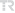## EXPLICIT FORMULAS FOR EXPONENTIAL OF 2 Chi 2 SPLIT-COMPLEX MATRICES

COMMUNICATIONS FACULTY OF SCIENCES UNIVERSITY OF ANKARA-SERIES A1 MATHEMATICS AND STATISTICS, vol.71, no.2, pp.518-532, 2022 (ESCI)• Publication Type: Article / Article
• Volume: 71 Issue: 2
• Publication Date: 2022
• Doi Number: 10.31801/cfsuasmas.991894
• Journal Name:
• Journal Indexes: Emerging Sources Citation Index (ESCI), TR DİZİN (ULAKBİM)
• Page Numbers: pp.518-532
• Keywords: Split-complex numbers, hyperbolic numbers, matrix exponential, DIAGONALIZATION
• Recep Tayyip Erdoğan University Affiliated: Yes

#### Abstract

Split-complex (hyperbolic) numbers are ordered pairs of real numbers, written in the form x + jy with j(2) - 1, used to describe the geometry of the Lorentzian plane. Since a null split-complex number does not have an inverse, some methods to calculate the exponential of complex matrices are not valid for split-complex matrices. In this paper, we examined the exponential of a 2 Chi 2 split-complex matrix in three cases : i. triangle = 0, ii. triangle not equal 0 and triangle is not null split-complex number, iii. triangle (sic) 0 and triangle is a null split-complex number where triangle = (trA)(2) - 4 det A.